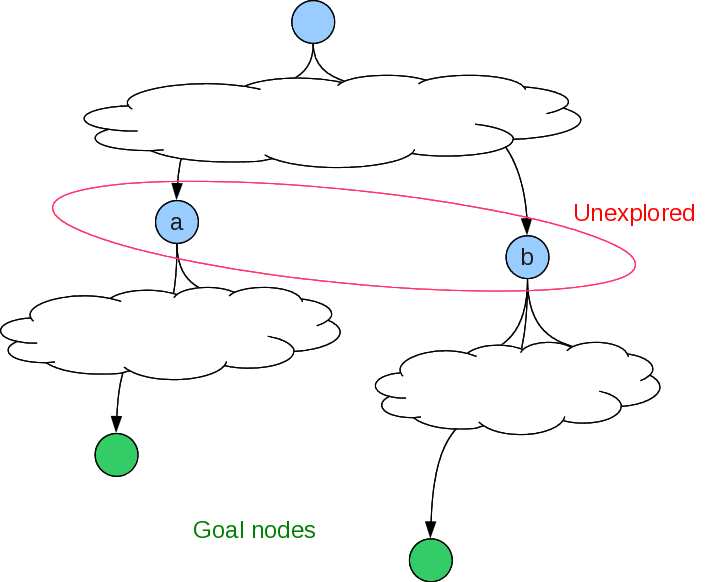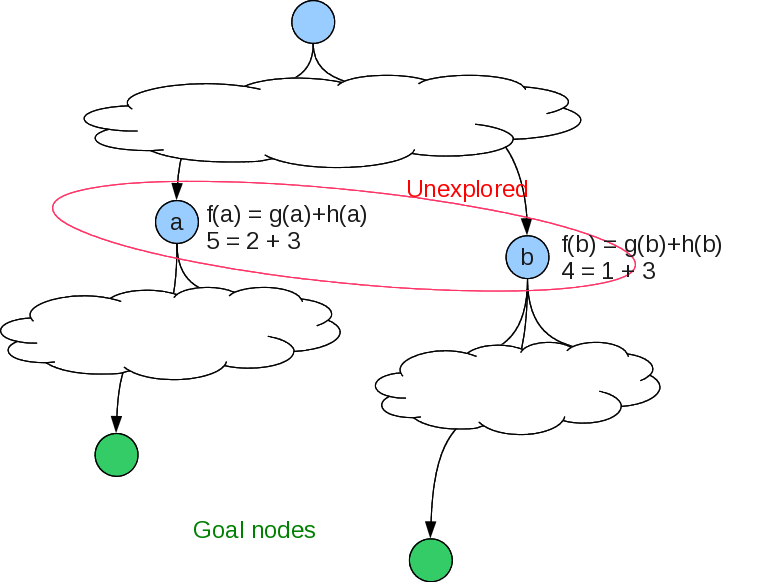{ "cells": [ { "cell_type": "markdown", "metadata": {}, "source": [ "# Informed Search" ] }, { "cell_type": "markdown", "metadata": {}, "source": [ "Also known as \"heuristic\" search, because the search is informed by an\n", "estimate of the total path cost through each node, and the next\n", "unexpanded node with the lowest estimated cost is expanded next. \n", "\n", " At some intermediate node, the \n", " estimated cost of the solution path =\n", " the sum of the step costs so far from the start node to this node\n", " +\n", " an estimate of the sum of the remaining step costs to a goal\n", "\n", "Let's label these as\n", "\n", " * \$f(n) =\$ estimated cost of the solution path through node \$n\$\n", " * \$g(n) =\$ the sum of the step costs so far from the start node to this node\n", " * \$h(n) =\$ an estimate of the sum of the remaining step costs to a goal\n", "\n", "*heuristic function*: \$h(n) =\$ estimated cost of the cheapest path from state at node \$n\$ to a goal state.\n", "\n", "\n", "\n", "Should we explore under Node a or b?\n", "\n", "" ] }, { "cell_type": "markdown", "metadata": {}, "source": [ "# A* algorithm" ] }, { "cell_type": "markdown", "metadata": {}, "source": [ "## Non-recursive" ] }, { "cell_type": "markdown", "metadata": {}, "source": [ "So, now you know enough python to try to implement A\\*, at least a non-recursive form. Start with your graph search algorithm from Assignment 1. Modify it so that the next node selected is based on its `f` value.\n", "\n", "For a given problem, define `start_state`, `actions_f`, `take_action_f`, `goal_test_f`, and a heuristic function `heuristic_f`. `actions_f` must return valid actions paired with the single step cost, and `take_action_f` must return the pair containing the new state and the cost of the single step given by the action. We can use the `Node` class to hold instances of nodes. However, since this is not a recursive algorithm, `Node` must be extended to include the node's parent node, to be able to generate the solution path once the search finds the goal.\n", "\n", "Now the A* algorithm can be written as follows\n", " * Initialize `expanded` to be an empty dictionary\n", " * Initialize `un_expanded` to be a list containing the start_state node. Its `h` value is calculated using `heuristic_f`, its `g` value is 0, and its `f` value is `g+h`.\n", " * If `start_state` is the `goal_state`, return the list containing just `start_state` and its `f` value to show the cost of the solution path.\n", " * Repeat the following steps while `un_expanded` is not empty:\n", " * Pop from the front of `un_expanded` to get the best (lowest f value) node to expand.\n", " * Generate the `children` of this `node`.\n", " * Update the `g` value of each child by adding the action's single step cost to this node's `g` value.\n", " * Calculate `heuristic_f` of each child.\n", " * Set `f = g + h` of each child.\n", " * Add the node to the `expanded` dictionary, indexed by its state.\n", " * Remove from `children` any nodes that are already either in `expanded` or `un_expanded`, unless the node in `children` has a lower f value.\n", " * If `goal_state` is in `children`:\n", " * Build the solution path as a list starting with `goal_state`. \n", " * Use the parent stored with each node in the `expanded` dictionary to construct the path.\n", " * Reverse the solution path list and return it.\n", " * Insert the modified `children` list into the `un_expanded` list and ** sort by `f` values.**" ] }, { "cell_type": "markdown", "metadata": {}, "source": [ "## Recursive" ] }, { "cell_type": "markdown", "metadata": {}, "source": [ "Our authors provide the Recursive Best-First Search algorithm, which\n", "is A\\* in a recursive, iterative-deepening form, where depth is now\n", "given by the \$f\$ value. Other differences from just\n", "iterative-deepening A\\* are:\n", " - depth-limit determined by \$f\$ value of best alternative to node being explored, so will stop when alternative at the node's level looks better;\n", " - \$f\$ value of a node is replaced by best \$f\$ value of its children, so any future decision to try expanding this node again is more informed.\n", "\n", "It is a bit difficult to translate their pseudo-code into python. Here is my version. Let's step through it." ] }, { "cell_type": "code", "execution_count": 32, "metadata": {}, "outputs": [ { "name": "stdout", "output_type": "stream", "text": [ "Overwriting a_star_search.py\n" ] } ], "source": [ "%%writefile a_star_search.py\n", "# Recursive Best First Search (Figure 3.26, Russell and Norvig)\n", "# Recursive Iterative Deepening form of A*, where depth is replaced by f(n)\n", "\n", "class Node:\n", "\n", " def __init__(self, state, f=0, g=0, h=0):\n", " self.state = state\n", " self.f = f\n", " self.g = g\n", " self.h = h\n", "\n", " def __repr__(self):\n", " return f'Node({self.state}, f={self.f}, g={self.g}, h={self.h})'\n", "\n", "def a_star_search(start_state, actions_f, take_action_f, goal_test_f, heuristic_f):\n", " h = heuristic_f(start_state)\n", " start_node = Node(state=start_state, f=0 + h, g=0, h=h)\n", " return a_star_search_helper(start_node, actions_f, take_action_f, \n", " goal_test_f, heuristic_f, float('inf'))\n", "\n", "def a_star_search_helper(parent_node, actions_f, take_action_f, \n", " goal_test_f, heuristic_f, f_max):\n", "\n", " if goal_test_f(parent_node.state):\n", " return ([parent_node.state], parent_node.g)\n", " \n", " ## Construct list of children nodes with f, g, and h values\n", " actions = actions_f(parent_node.state)\n", " if not actions:\n", " return ('failure', float('inf'))\n", " \n", " children = []\n", " for action in actions:\n", " (child_state, step_cost) = take_action_f(parent_node.state, action)\n", " h = heuristic_f(child_state)\n", " g = parent_node.g + step_cost\n", " f = max(h + g, parent_node.f)\n", " child_node = Node(state=child_state, f=f, g=g, h=h)\n", " children.append(child_node)\n", " \n", " while True:\n", " # find best child\n", " children.sort(key = lambda n: n.f) # sort by f value\n", " best_child = children\n", " if best_child.f > f_max:\n", " return ('failure', best_child.f)\n", " # next lowest f value\n", " alternative_f = children.f if len(children) > 1 else float('inf')\n", " # expand best child, reassign its f value to be returned value\n", " result, best_child.f = a_star_search_helper(best_child, actions_f,\n", " take_action_f, goal_test_f,\n", " heuristic_f,\n", " min(f_max,alternative_f))\n", " if result != 'failure': # g\n", " result.insert(0, parent_node.state) # / \n", " return (result, best_child.f) # d\n", " # / \\ \n", "if __name__ == \"__main__\": # b h \n", " # / \\ \n", " successors = {'a': ['b','c'], # a e \n", " 'b': ['d','e'], # \\ \n", " 'c': ['f'], # c i\n", " 'd': ['g', 'h'], # \\ / \n", " 'f': ['i','j']} # f \n", " # \\\n", " def actions_f(state): # j \n", " try:\n", " ## step cost of each action is 1\n", " return [(succ, 1) for succ in successors[state]]\n", " except KeyError:\n", " return []\n", "\n", " def take_action_f(state, action):\n", " return action\n", "\n", " def goal_test_f(state):\n", " return state == goal\n", "\n", " def h1(state):\n", " return 0\n", "\n", " start = 'a'\n", " goal = 'h'\n", " result = a_star_search(start, actions_f, take_action_f, goal_test_f, h1)\n", "\n", " print(f'Path from a to h is {result} for a cost of {result}')" ] }, { "cell_type": "markdown", "metadata": {}, "source": [ "Running this shows" ] }, { "cell_type": "code", "execution_count": 33, "metadata": {}, "outputs": [ { "name": "stdout", "output_type": "stream", "text": [ "Path from a to h is ['a', 'b', 'd', 'h'] for a cost of 3\n" ] } ], "source": [ "run a_star_search.py" ] }, { "cell_type": "code", "execution_count": 34, "metadata": {}, "outputs": [ { "data": { "text/plain": [ "[('b', 1), ('c', 1)]" ] }, "execution_count": 34, "metadata": {}, "output_type": "execute_result" } ], "source": [ "actions_f('a')\n", "valid_ones = actions_f('a')\n", "valid_ones" ] }, { "cell_type": "code", "execution_count": 35, "metadata": {}, "outputs": [ { "data": { "text/plain": [ "('b', 1)" ] }, "execution_count": 35, "metadata": {}, "output_type": "execute_result" } ], "source": [ "take_action_f('a', valid_ones)" ] }, { "cell_type": "markdown", "metadata": {}, "source": [ "Actually, there is in error in this code. Try using it to search for a goal that does not exist!" ] } ], "metadata": { "anaconda-cloud": {}, "jupytext": { "formats": "ipynb,py:light" }, "kernelspec": { "display_name": "Python 3", "language": "python", "name": "python3" }, "language_info": { "codemirror_mode": { "name": "ipython", "version": 3 }, "file_extension": ".py", "mimetype": "text/x-python", "name": "python", "nbconvert_exporter": "python", "pygments_lexer": "ipython3", "version": "3.8.3" }, "toc": { "base_numbering": 1, "nav_menu": {}, "number_sections": true, "sideBar": true, "skip_h1_title": false, "title_cell": "Table of Contents", "title_sidebar": "Contents", "toc_cell": false, "toc_position": {}, "toc_section_display": true, "toc_window_display": false } }, "nbformat": 4, "nbformat_minor": 1 }